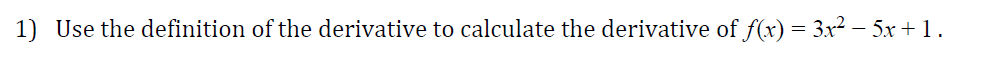# 1)Use the definition of the derivative to calculate the derivative off(x) = 3x2-5x+ 1

Question
1 viewshelp_outlineImage Transcriptionclose1) Use the definition of the derivative to calculate the derivative off(x) = 3x2-5x+ 1 fullscreen
check_circle

Step 1

Find the derivative of f (x) = 3x2 –5x +1.

Step 2

Definition of derivative:

Step 3

The given function is f (x) = 3x2 –5x +1. Then f (x + h) = 3(x + h)2 –5(x +...

### Want to see the full answer?

See Solution

#### Want to see this answer and more?

Solutions are written by subject experts who are available 24/7. Questions are typically answered within 1 hour.*

See Solution
*Response times may vary by subject and question.
Tagged in

### Derivative